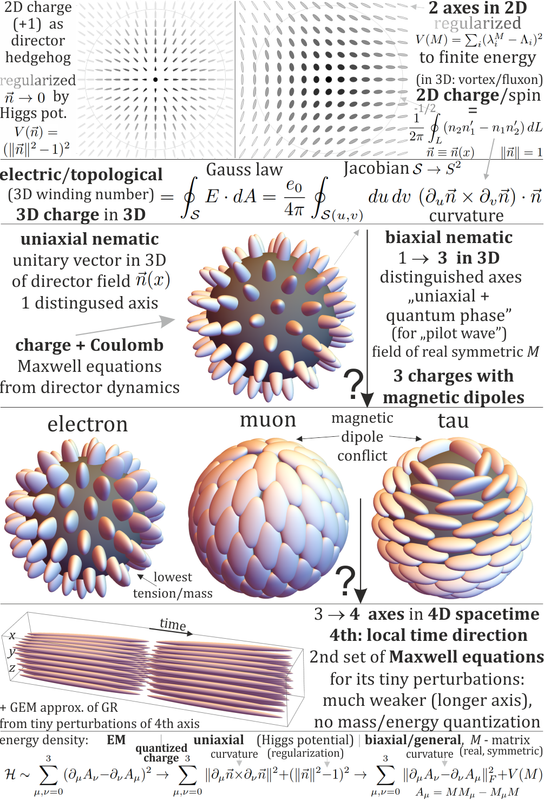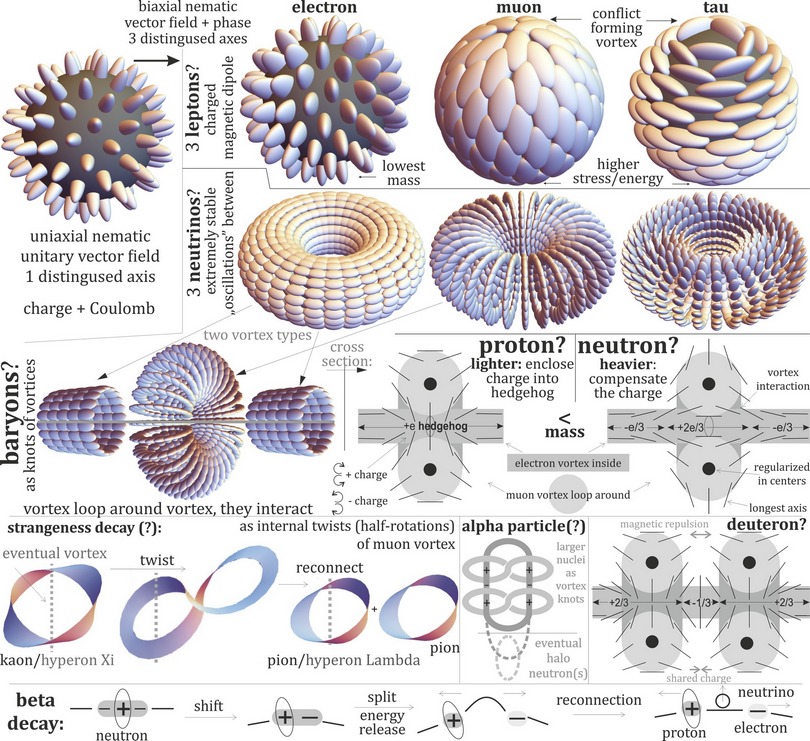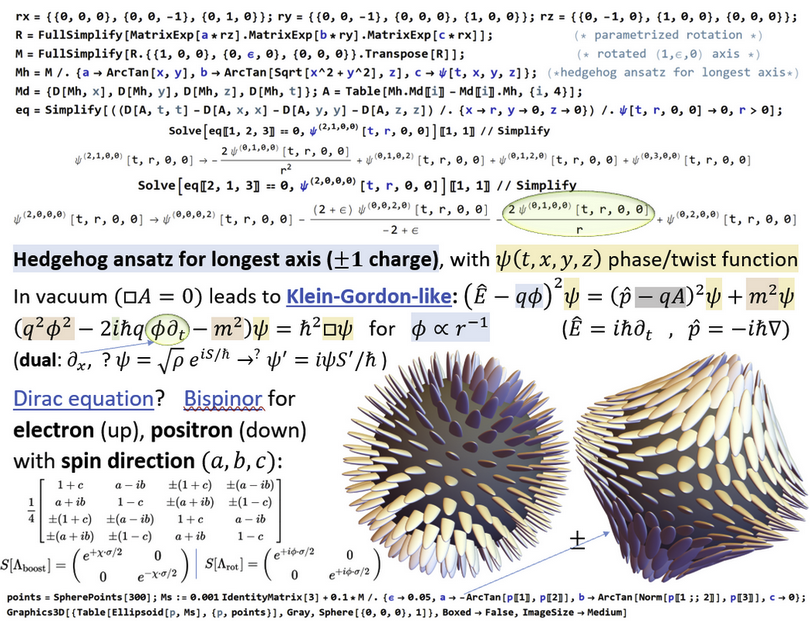## EM+gravity framework for (quantized) topological charges

Foundations of physics and/or philosophy of physics, and in particular, posts on unresolved or controversial issues

### EM+gravity framework for (quantized) topological charges

I have finally worked out mathematical framework up to Euler-Lagrange for this ellipsoid field/liquid crystal like approach: https://arxiv.org/pdf/2108.12359
- field of 3 distinguishable axes using 3x3 matrices preferring fixed set of eigenvalues,
- hedgehog of one of 3 axes for 3 leptons - charges governed by Maxwell equations, with magnetic dipole moment due to hairy ball theorem,
- then expanding to 4x4 matrices we get second set of Maxwell equations for GEM ( https://en.wikipedia.org/wiki/Gravitoelectromagnetism ).
They experimentally get long-range e.g. Coulomb-like interaction for topological charges in liquid crystals (e.g. https://www.nature.com/articles/s41598-017-16200-z ) - we can expand it to further particles and gravity.

The approach generalizes Faber's from vector to matrix field.
Electromagnetic (A vector, F tensor) are no longer just (connection Gamma, curvature R), but additionally include dependence of rotated shape (eigenvalues).
This way we can get vacuum dynamics of 3 strengths: EM >> pilot wave >> GEM.Jarek

Posts: 241
Joined: Tue Dec 08, 2015 1:57 am

### Re: EM+gravity framework for (quantized) topological charges

Very nice illustrations but not exactly sure what your overall main point is?
.
FrediFizzx
Independent Physics Researcher

Posts: 2905
Joined: Tue Mar 19, 2013 7:12 pm
Location: N. California, USA

### Re: EM+gravity framework for (quantized) topological charges

One point is that I agree with many people here that Bell theorem is not a problem (however, with a different argumentation) - so shouldn't the next step be searching for details of microscopic physics, like particle models?
So where to search for such models?
Maybe in hydrodynamics?

There are lots of hydrodynamical QM analogs e.g. Casimir, Aharonov-Bohm, double-slits interference, tunneling, orbit quantization (including Zeeman, double quantization), recreating quantum statistics - some gathered: https://www.dropbox.com/s/kxvvhj0cnl1iqxr/Couder.pdf
Especially in superconductors/superfluids there are observed so called macroscopic quantum phenomena ( https://en.wikipedia.org/wiki/Macroscop ... _phenomena ) - stable configurations like fluxon/Abrikosov vortex quantizing magnetic field due to topological constraints (phase change along loop has to be multiplicity of 2pi).
There is observed e.g. interference ( https://journals.aps.org/prb/abstract/1 ... .85.094503 ), tunneling ( https://journals.aps.org/prb/pdf/10.110 ... B.56.14677 ),
Also there is this famous Volovik's "The universe in helium droplet" book ( http://www.issp.ac.ru/ebooks/books/open ... roplet.pdf ).

Another point is just repairing electromagnetism:
1) that Gauss law can return any real charge, while in nature only integer - it can repaired by interpreting field curvature as electric field, making that Gauss law counts topological charge.
2) that point charge has infinite energy of electric field - what is repaired here by using Higgs-like potentials, allowing to deform field to prevent this infinity.

Finally, it seems at least interesting that looking at liquid crystals, its menagerie of topological defects nicely resembles particle menagerie:Jarek

Posts: 241
Joined: Tue Dec 08, 2015 1:57 am

### Re: EM+gravity framework for (quantized) topological charges

Prepared slides with lots of materials about this resemblance between liquid crystal topological defects and particle physics:
https://www.dropbox.com/s/9dl2g9lypzqu5 ... ticles.pdf
Jarek

Posts: 241
Joined: Tue Dec 08, 2015 1:57 am

### Re: EM+gravity framework for (quantized) topological charges

Some updates - interactive demonstration to play with such topological charges of liquid crystal biaxial nematic - of 3 types resembling 3 leptons, requiring magnetic dipoles, with analogy of quantum phase evolution: https://demonstrations.wolfram.com/Topo ... idCrystal/

Derivation of Klein-Gordon-like equation for this evolving phase (slide 15 of https://www.dropbox.com/s/9dl2g9lypzqu5hp/liquid%20crystal%20particles.pdf):Jarek

Posts: 241
Joined: Tue Dec 08, 2015 1:57 am